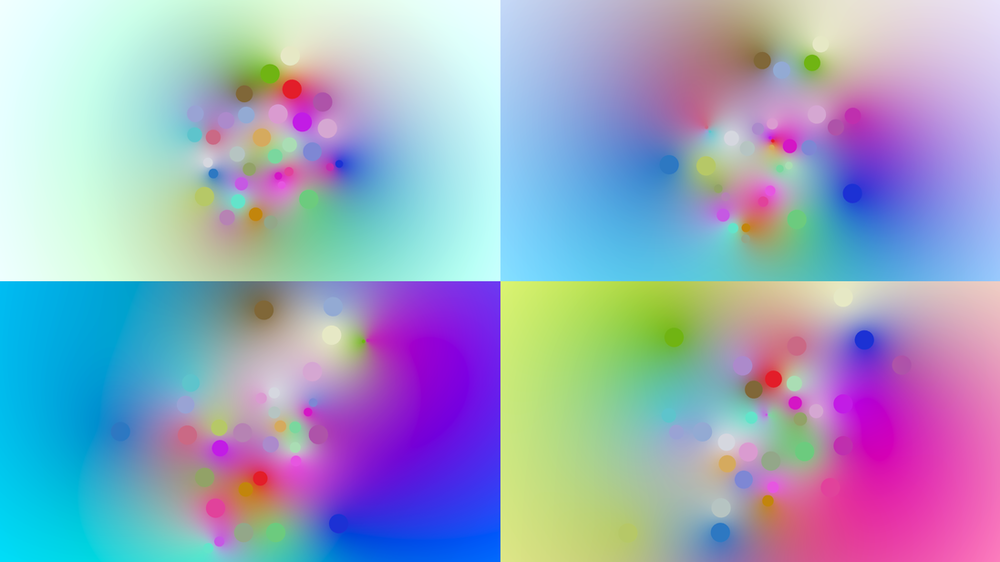# Create a Landscape from Irregular Points using Multivariate Interpolation

## Recommended Posts

Posted (edited)

This is an example for building landscapes from irregular points using SciPy.

```import numpy as np
from scipy.interpolate import griddata

# FIRST INPUT: REGULAR GRID
node = hou.pwd()
geo1 = node.geometry()

grid_x = np.array(geo1.pointFloatAttribValues("px"))
grid_y = np.array(geo1.pointFloatAttribValues("py"))
grid_z = np.array(geo1.pointFloatAttribValues("pz"))

# SECOND INPUT: RANDOM POINTS
inputs = node.inputs()
geo2 = inputs.geometry()

val_x = np.array(geo2.pointFloatAttribValues("px"))
val_y = np.array(geo2.pointFloatAttribValues("py"))
val_z = np.array(geo2.pointFloatAttribValues("pz"))

# MULTIVARIATE INTERPOLATION
grid_y = griddata( (val_x, val_z), val_y, (grid_x, grid_z), fill_value=0.0, method='cubic' ) # methods: nearest, cubic, linear

# NEW HEIGHT POSITIONS ON GRID
geo1.setPointFloatAttribValuesFromString("py",grid_y.astype(np.float32))```
Edited by konstantin magnus
•1

##### Share on other sites

I just found out about the radial basis function (RBF) in Scipy.interpolate, which does an even better job at interpolating between irregular points. As it does not use delaunay, it also lost the trianglish appearance it had before. RBF looks way smoother than what you´d get from Attribute Transfer SOP, too.Btw: Does someone know, how to make the same code work with vector arrays in NumPy?

I tried it in the commented lines, but currently I have to use three separate arrays for each color channel and therefore call each function three times, as well : (

```import numpy as np
from scipy.interpolate import griddata
import scipy.interpolate as interp

node = hou.pwd()
geo1 = node.geometry()

inputs = node.inputs()
geo2 = inputs.geometry()

method_nr = node.evalParm('method')
method_str = method_names[method_nr]

grid_x = np.array(geo1.pointFloatAttribValues('px'))
grid_z = np.array(geo1.pointFloatAttribValues('pz'))

color_r = np.array(geo2.pointFloatAttribValues('cr'))
color_g = np.array(geo2.pointFloatAttribValues('cg'))
color_b = np.array(geo2.pointFloatAttribValues('cb'))
#color = np.array(geo2.pointFloatAttribValues('Cd'))
#np.reshape(color, (30, 3))

pos_x = np.array(geo2.pointFloatAttribValues('px'))
pos_z = np.array(geo2.pointFloatAttribValues('pz'))

rbf_red = interp.Rbf(pos_x, pos_z, color_r, function=method_str)
rbf_green = interp.Rbf(pos_x, pos_z, color_g, function=method_str)
rbf_blue = interp.Rbf(pos_x, pos_z, color_b, function=method_str)
#rbf_color = interp.Rbf(pos_x, pos_z, color, function=method_str)

smooth_rbf_red = rbf_red(grid_x, grid_z)
smooth_rbf_green = rbf_green(grid_x, grid_z)
smooth_rbf_blue = rbf_blue(grid_x, grid_z)
#smooth_rbf_color = rbf_color(grid_x, grid_z)

geo1.setPointFloatAttribValuesFromString("clr_r", smooth_rbf_red.astype(np.float32))
geo1.setPointFloatAttribValuesFromString("clr_g", smooth_rbf_green.astype(np.float32))
geo1.setPointFloatAttribValuesFromString("clr_b", smooth_rbf_blue.astype(np.float32))
#geo1.setPointFloatAttribValuesFromString("Cd", smooth_rbf_color.astype(np.float32))```

scipy_grid_to_color_points.hiplc

•2

## Create an account

Register a new account